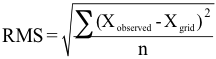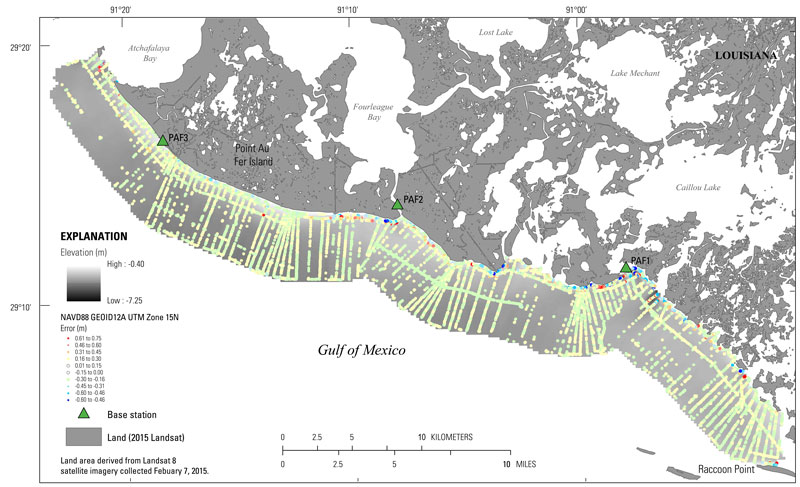Data Series 1041

### Coastal Single-Beam Bathymetry Data Collected in 2015 from Raccoon Point to Point Au Fer Island, Louisiana

 Citation Page Abstract Introduction Survey Overview Data Acquisition Data Processing Error Analysis Survey Products Data Downloads Logs Abbreviations References Cited

## Error Analysis

### Digital Elevation Model (DEM) Error

In order to evaluate how well the DEM represented the final sounding data, a comparison of the DEM cell value versus the final point elevations were plotted in ArcGIS using the “extract values by points” spatial analyst tool. This tool extracts the value represented by the underlying grid and compares it to that of the overlying point data. The root mean square (RMS) error, quantified as the difference between the measured depth and the interpolated depth values, was calculated for each platform using the following equation, where “X” represents the elevation value of the individual data point and “n” is the number of point elevations represented by the grid:There are a total of 4,483,280 point elevations represented by the grid, and the root mean square error of all points is 0.16 m. Eighty three percent of the point data were within ±0.15 m of the grid value; RMS error for these points is 0.06 m. To represent the spatial distribution of grid error, the difference between the DEM and the original sounding values were plotted, color coded, and overlaid on the DEM (fig. 8).Figure 8. Error values representing the difference between the Digital Elevation Model (DEM) and the value at each sample point. Points with grid error of +/-0.15 meters (m) were made transparent. [Click figure to enlarge]U.S. Department of the Interior | U.S. Geological Survey
URL: https://pubs.usgs.gov/ds/1041/ds1041_error-analysis.html